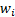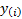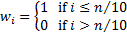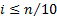/

### Extreme Events – Specimen Question A.2.1(e) – Answer/Hints

Q. What other methodologies could you use to formulate a view about how fat-tailed this return series might be if your focus was principally on fat-tailed behaviour around or below the lower 10th percentile quantile level?

With a sample size of 20 there are only two data points in the sample around or below the lower 10th percentile quantile level. This is too few to permit any meaningful statistical analysis to be carried out in this instance.

However, if the sample size were much larger, then we could carry out the following:

(a)    We could define a weighting ‘schema’, i.e.,  to apply to the ordered data series,. In this case the form of the question might lead us to use:(b)   We could calculate the normal distribution that best fitted the observed data sample, but giving weightto the observations, i.e. here only taking into account data where. To do so we would use functions as set out in the Nematrian web page on Weighted Moments and Cumulants.

(c)    We could now compare the ordered observed data versus that ‘expected’ were the normal distribution in (ii) to have applied, perhaps visually and/or perhaps fitting suitable curves through this comparison, e.g. a variant of the approach  underling the Nematrian’s standard weighted cubic curve fit.

Formal tests for non-normality could then be carried out using suitable refinements to standard test methodologies, see TestsForNormality.